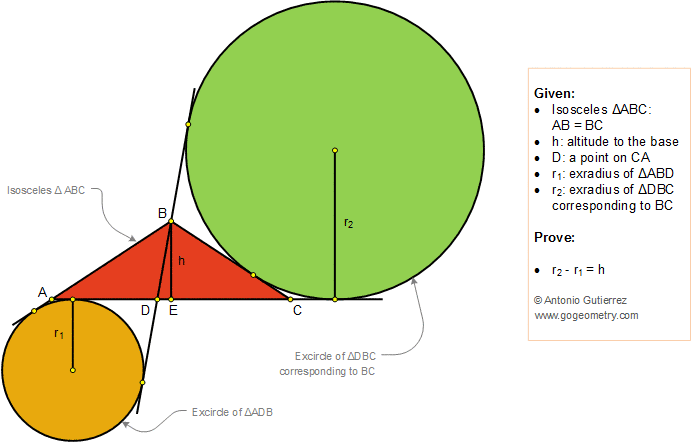# Geometry Problem 1375: Isosceles Triangle, Interior Cevian, Exradius, Excircle, Altitude to the Base.

### Proposition

In the figure below, ABC is an isosceles triangle (AB = BC) with altitude BE = h. D is a point on AC, r1 is the exradius of the triangle ABD corresponding to AD and r2 is the exradius of the triangle DBC corresponding to BC. Prove that r2 - r1 = h.

BD is called an interior cevian of triangle ABC.